# ICLR 2020 | 抛开卷积，multi-head self-attention能够表达任何卷积操作

2019/04/10 10:10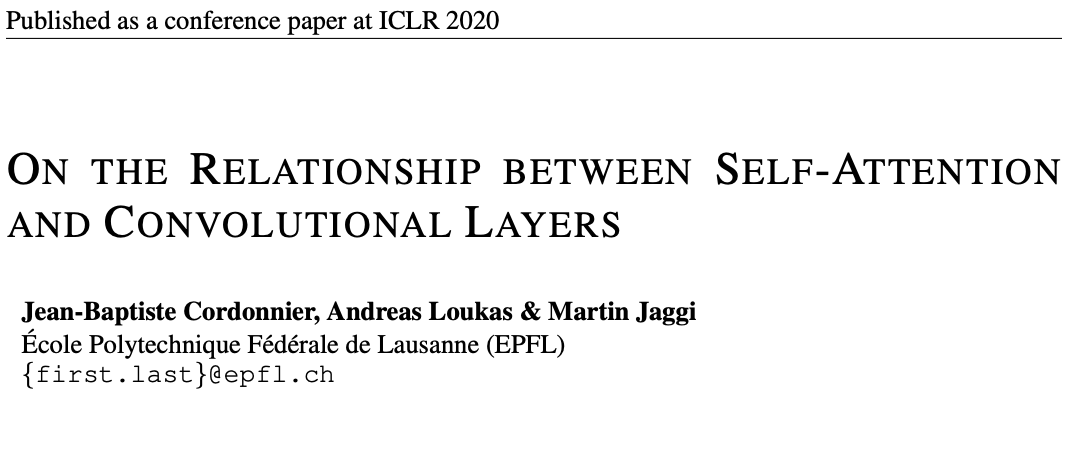# Introduction

transformer的提出对NLP领域的研究有很大的促进作用，得益于attention机制，特别是self-attention，会考虑词间的相似性，对当前词进行加权输出。受到词间关系学习的启发，self-attention也开始用于视觉任务中，但大都是attention和convonlution的结合。Ramachandran在19年的研究中，用full attention model达到了resnet baseline的精度，模型参数和计算量相比卷积网络减轻了不少
因此，论文主要研究self-attention layer在图片处理上是否能达到convolutional layer的效果，贡献如下：

• 在理论层面，论文通过构造性证明self-attention layers能够替代任何卷积层

# Background on attention mechanisms for vision

定义$X\in \mathbb{R}^{T\times D_{in}}$为输入矩阵，包含$T$个$D_{in}$维的token，在NLP中，token对应着序列化的词，同样地也可以对应序列化的像素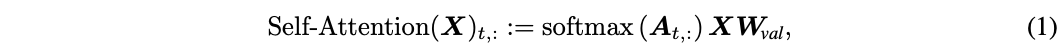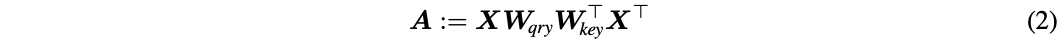self-attention layer从$D_{in}$到$D_{out}$的计算如公式1,2所示，$A$为attention scores，softmax将score转换为attention probabilities，该层的参数包含查询矩阵(query matrix)$W_{qry}\in \mathbb{R}^{D_{in}\times D_k}$，关键词矩阵(key matrix)$W_{key}\in \mathbb{R}^{D_{in}\times D_k}$，值矩阵(value matrix)$W_{val}\in \mathbb{R}^{D_{in}\times D_{out}}$，都用于对输入进行变化，基本跟NLP中的self-attention一致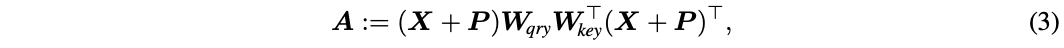因为只考虑相关性，self-attention一个很重要的属性是，不管输入的顺序如何改变，输出都是不变的，这对于希望顺序对结果有影响的case影响很大，因此在self-attention基础上为每个token学习一个positional encoding参数，$P\in \mathbb{R}^{T\times D_{in}}$为包含位置信息的embedding向量，可以有多种形式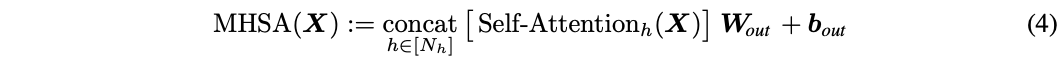这里采用multiple heads版本的self-attention，每个head的参数矩阵都不一样，能够提取不同的特征，$N_h$个head输出$D_h$维结果concat后映射成$D_{out}$维的最终输出，两个新参数，映射矩阵(projection matrix)$W_{out}\in \mathbb{R}^{N_hD_h\times D_{out}}$，偏置$b_{out}\in \mathbb{R}^{D_{out}}$

### Attention for images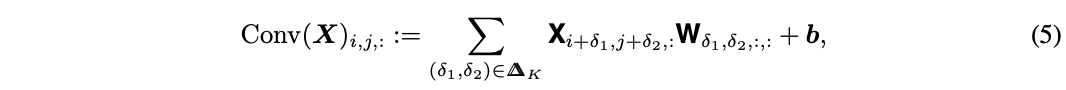卷积是最适合神经网络的图片操作方法，给予图片$X\in \mathbb{R}^{W\times H\times D_{in}}$，卷积在$(i,j)$的操作如公式5，$W\in \mathbb{R}^{K\times K\times D_{in}\times D_{out}}$，$b\in \mathbb{R}^{D_{out}}$，K为卷积核的大小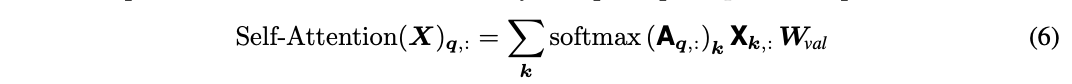在图片上应用self-attention，定义查询像素和关键词像素$q,k\in[W]\times [H]$，输入的向量大小为$X\in \mathbb{R}^{W\times H\times D_{in}}$为了保持一致性，用1D的符号来代表2D坐标，比如$p=(i,j)$，用$X_p$代表$X_{ij}$，用$A_p$代表$A_{ij}$

### Positional encoding for images

位置编码目前主要有两种，分别是绝对位置(absolute)编码和相对(relative)位置编码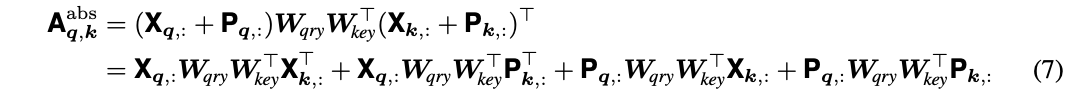在绝对位置编码中，每个像素拥有一个位置向量$P_p$(学习的或固定的)，于是公式2可以转换为公式7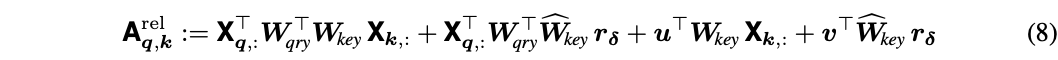相对位置编码的核心是只考虑查询像素和查询像素之间的位置差异，如公式8，大体是将公式7的每一项的绝对位参数改为相对位置参数。attention scores只跟偏移$\delta:=k-q$，$u$和$v$是learnable参数，每个head都不一样，而每个偏移的相对位置编码$r_\delta\in \mathbb{R}^{D_p}$是head共享的。关键词权重分成了两部分，$W_{key}$属于输入，$\widehat {W}_{key}$属于偏移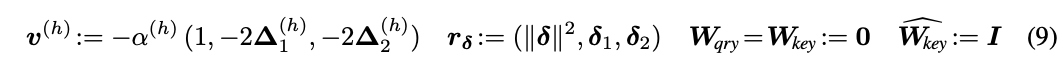公式9称为二次编码(quadratic encoding)，参数$\Delta^{(h)}=(\Delta_1^{(h)},\Delta_2^{(h)})$和$\alpha^{(h)}$分别代表中心点以及attention区域的大小，都是通过学习得来的，而$\delta=(\delta_1,\delta_2)$则是固定的，代表查询像素和关键词像素的相对位移

# Self-attention as a convolutional layer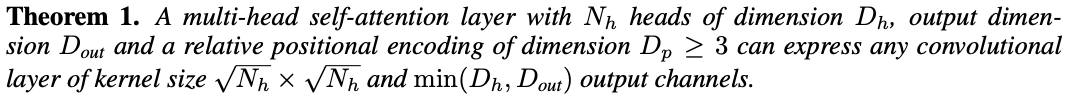定理1，对于multi-head self-attention，$N_h$个head，每个head输出$D_h$维，整体最终输出$D_{out}$，相对位置编码$D_p\ge 3$维，可以表示任何卷积，核大小为$\sqrt{N_h}\times \sqrt{N_h}$，output channel为$min(D_h,D_{out})$
对于output channel不是固定$D_{out}$，论文认为当$D_h<D_{out}$时，$W_{out}$相当于一个升维操作，这个操作的特征提取不能代表原始卷积的属性，实际中，一般采用$D_h=D_{out}，$这里是我的个人理解，可能有错误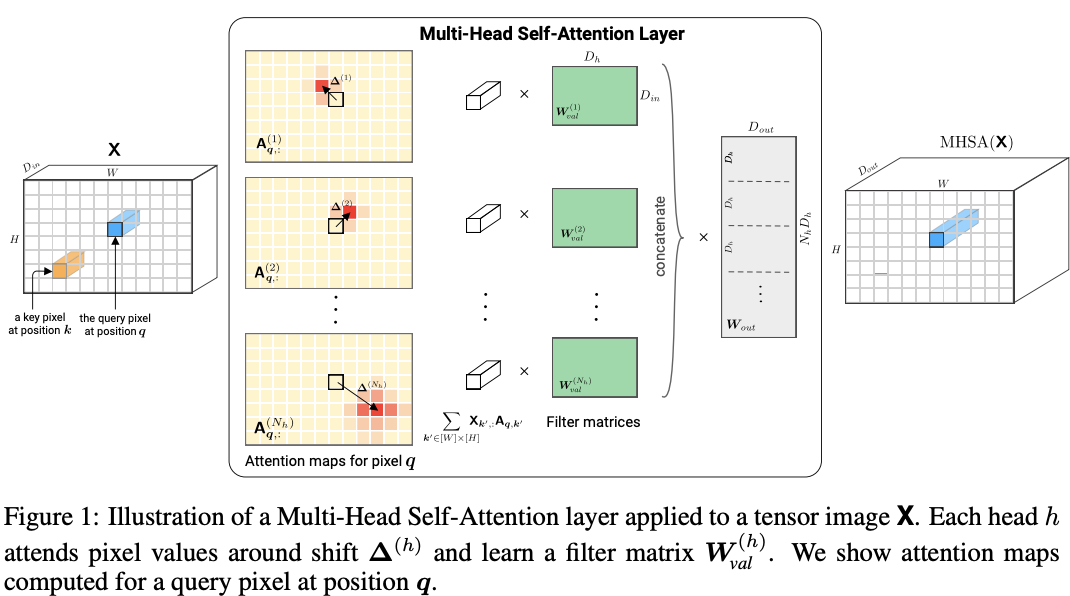上面的定理表明，在选择适当的参数后，multi-head self-attention layer可以表现得跟卷积层一样，每个head的attention score关注不同偏移距离的像素，偏移值分别在集合$\Delta_K={-\lfloor K/2\rfloor,...,\lfloor K/2\rfloor}$内，这样整体就类似于$K\times K$核，如图1所示
卷积神经网络不止卷积核大小这个超参，还有很多其它超参，这里论文对输出的数值的一致性上进行了解释：

• Padding: multi-head self-attention layer默认使用"SAME"的填充模式，而卷积层会减小K-1个像素的图片大小，因此，为了减少边界影响，可以对卷积图片进行$\lfloor K/2\rfloor$的零填充
• Stride: 卷积神经网络的步长可以认为是在卷积后面加入一个pooling操作，而Theorem 1默认步长为1，但可以在后面接个pooling达到相同的结果

# Experiments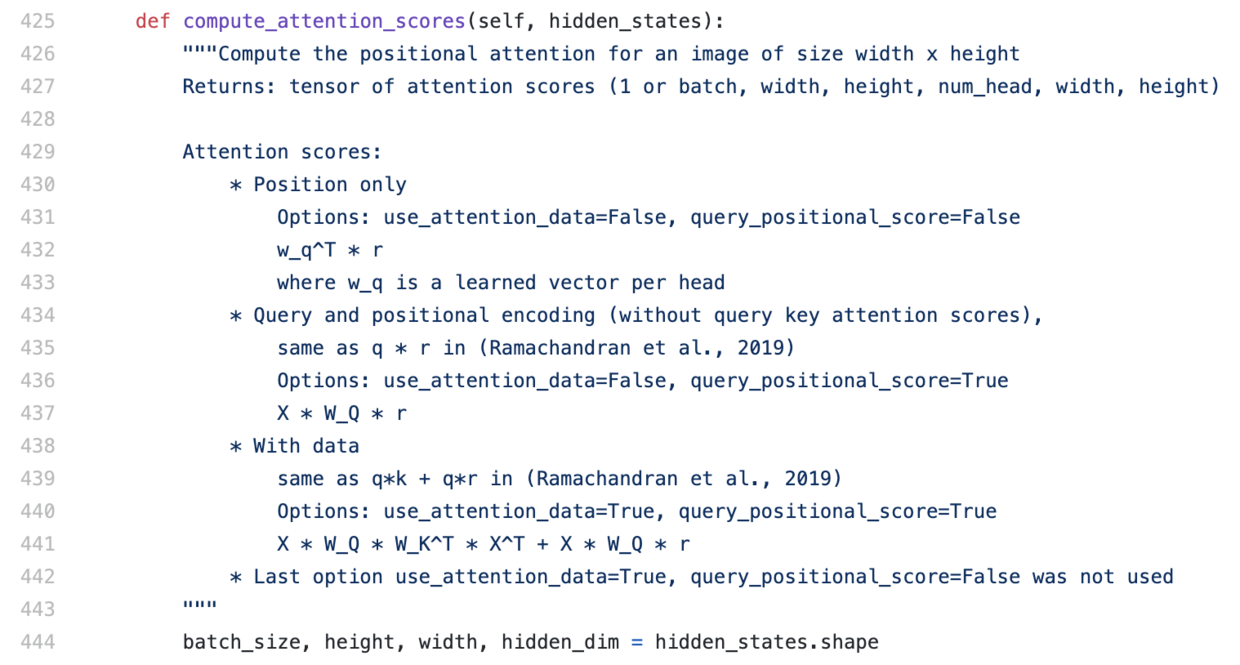实验的主要目的在于验证self-attention进行类似卷积的操作，以及self-attention在实际中是否学习到了类似卷积的属性，包含3种类似的attention，分别为quadratic embedding，learned embedding和learnded embedding + content，具体可以看作者开源代码的attention score计算部分。根据作者的解答，前两种都对应于代码的第一种注释，区别在前者按照公式9来计算$r_{\delta}$，后者则是完全随机学习来的，最后一种则对应注释的第三种

### Implementation details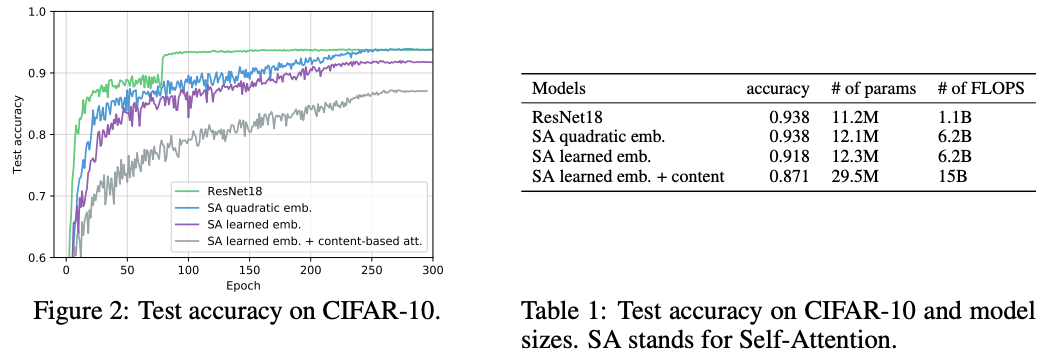结果如图2和Table1所示，ResNet收敛更快，但不能确定这是卷积固有的属性还是结构优化带来的结果，由于实验的结构还是很naive的，所以会存在差距，通过一些优化手段应该可以解决

论文进行实验验证公式9的相对位置编码$r_{\delta}$是否学习到了类似卷积的操作，实验使用9个head来模拟$3\times 3$卷积操作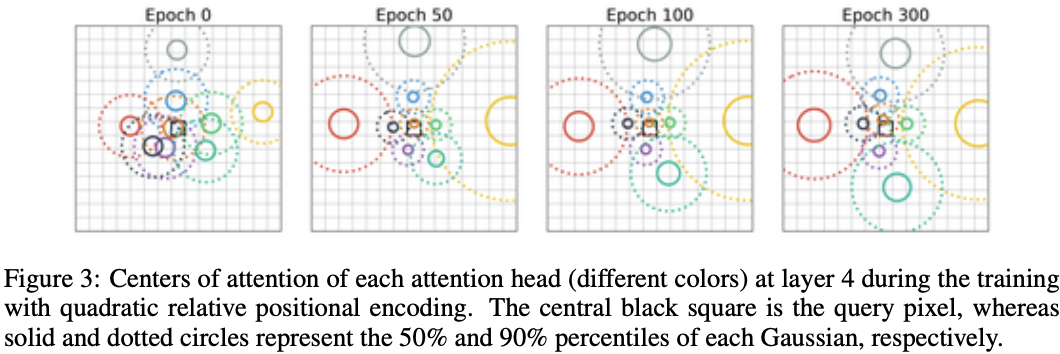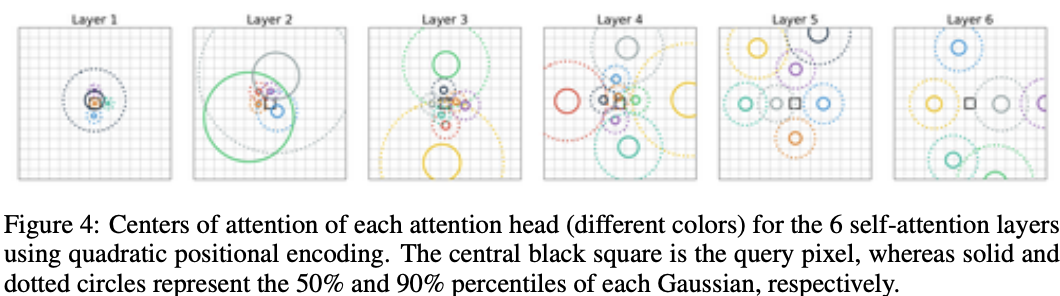### Learned relative positional encoding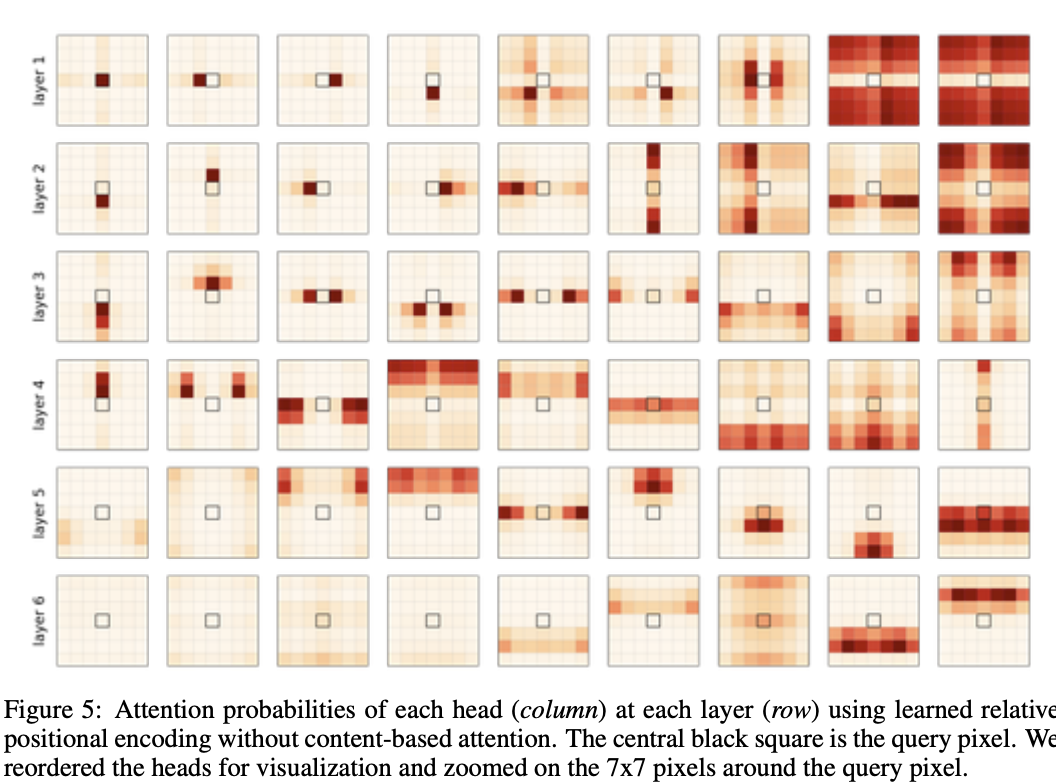首先，论文去除了与输入数据相关的attention内容，仅考虑公式8的最后一项进行统计，结果如图5所示，层1-3非常接近查询区域，而深层数据则更关注整图的信息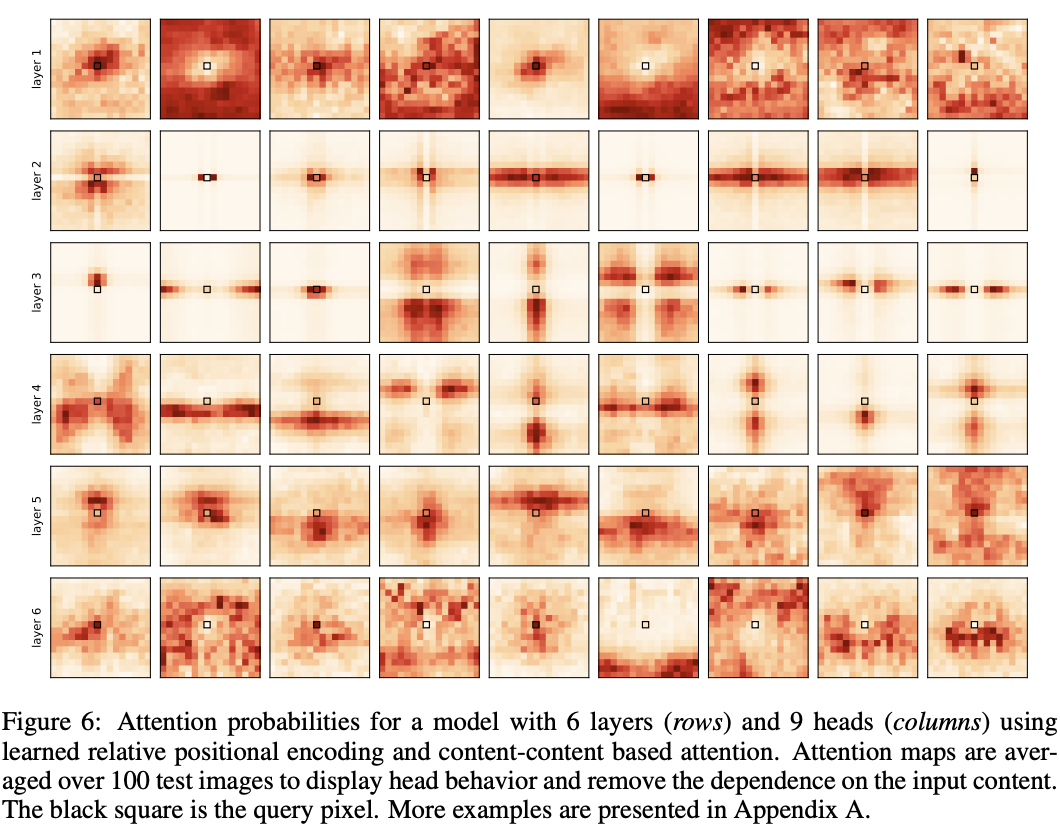接着使用论文对positional attention和content-based attention进行了分析$(q^\intercal r+q^\intercal k)$，将100张图的attention probabilities进行了平均结果如图6所示。在层2和3中，尽管输入的数据不一样，但一些head学到了去关注查询像素附近的特定区域的像素这一行为，与卷积操作十分类似，而其它的head则使用了更多的content-based attention。在实际中，图6中的localized attention patterns是随着查询像素移动的，这与卷积操作更加类似，想请可以看 https://epfml.github.io/attention-cnn/

# CONCLUSION

论文展示了self-attention layers可以表示任意convolutional layer的行为，以及full-attentional模型能够学会如何结合local behavior和基于输入内容global attention。在未来，可以将卷积网络的优化方法迁移到full-attention模型上，应用于不同数据领域的模型，例如图像，文字和时间序列

### 感谢

在论文阅读期间遇到了很多问题，给作者发了邮件后，很耐心地回答了我的问题，在这里十分感谢作者Jean-Baptiste Cordonnier

### 参考内容0
0 收藏

### 作者的其它热门文章0 评论
0 收藏
0